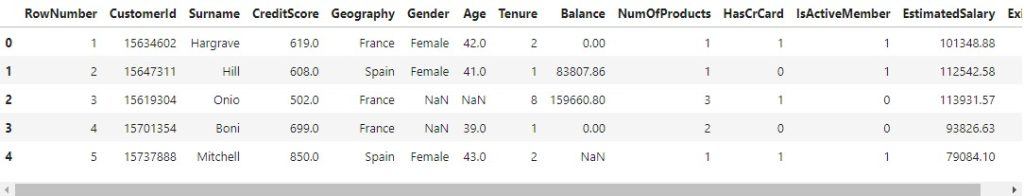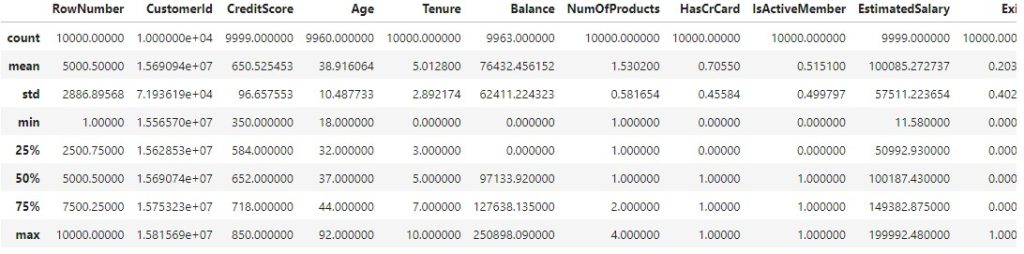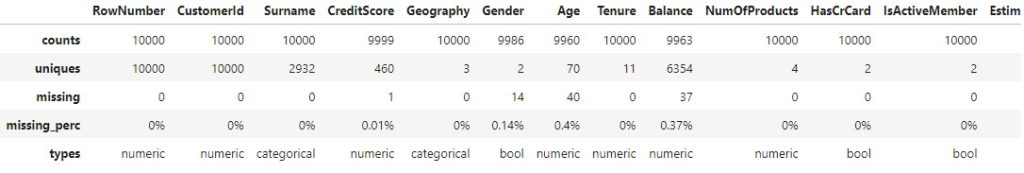# ML Modeling – Problem statement and Data description

ML modeling is the step where machine learning is used to find patterns in data and use that learned knowledge to predict an outcome. The type of ML modeling we are going to solve in this problem is called ‘Churn Modeling’.

Let’s first understand the Churn modeling problem statement and then go over the data we will use for this.

## What is Churn modeling?

Businesses want more customers. Not just that, they also want to retain their existing customers. In an open market, companies often compete to win over customers. In this process, they want to know which of their existing customers are likely going to attrite (stop buying) in the future, so they can make efforts to prevent that from happening.

This can be answered using Churn Modeling.

Churn modeling uses machine learning to predict the probability of Churn for a given customer.

## Data Description

In this example, we have a bank that wants to know who amongst their existing customers is going to churn. In the below dataset, each row represents an individual customer.

The ‘Exited’ column in the far right says if the customer ‘Exited’ (churned) or not. The remaining columns such as ‘CreditScore’, ‘Age’, ‘Tenure’, ‘Balance’, ‘IsActiveMember’ etc may contain patterns that can used to predict if the customer ‘Exited’ or not.

The ‘Exited’ column is called the response variable and the remaining columns are the ‘explanatory’ or ‘predictor’ variables.

The key thing here, is the predictor variables will be useful only if it contains information that can help explain/predict the response variable.

Since we are trying to predict a variable (‘Exited’), this is considered as a predictive modeling problem. Also, since ‘Exited’ is a categorical variable (values are either 1 or 0), it is considered as a classification problem.

The first step in your project is to:
1. Setup the necessary packages
3. Describe it

Install the necessary ML packages

We will be using 3 additional packages for ML: (i) xgboost (ii) lightgbm (iii) imbalanced-learn.

We haven’t installed them earlier. So, let’s do that now.

You can run pip install from inside jupyterlab instead of the Terminal by adding ! before the command. That way, jupyter will know it has to be run at the command line instead of being treated as a Python command.

!pip install xgboost
!pip install lightgbm
!pip install imbalanced-learn


Note: You can run the above commands in Jupyter lab or in Anaconda prompt / Terminal directly. If you run it in Jupyter lab, you need to add the ! before the command to let jupyter know that it should be sent to the terminal instead of running it as a Pyhon command. You may remove the ! if you run directly in the terminal.

## Import the packages

Don’t worry about the syntax now.

Just observe, we use 'import' statement followed by the names of packages we will be using. Often we given aliases to package names, like pandas as pd, numpy as np. This is purely to reduce the number of characters to type, more of a coding convention.

Let’s packages for data manipulation (Numpy, pandas), visualization (matplotlib), machine learning (sckit-learn, xgboost, lightgbm) etc.

There will be more packages needed later on for specific cases. We will install them later. But this list you see here is kinda standard, commonly used packages.

# Import libraries

# Data Manipulation
import numpy as np
import pandas as pd
from   pandas import DataFrame

# Data Visualization
import seaborn as sns
import matplotlib.pyplot as plt

# Machine Learning
from   sklearn.preprocessing import LabelEncoder, StandardScaler, OrdinalEncoder
from   sklearn.impute import SimpleImputer
from   sklearn.model_selection import train_test_split, GridSearchCV
from   sklearn.metrics import confusion_matrix , classification_report, accuracy_score, roc_auc_score, plot_roc_curve
from   sklearn.linear_model import LogisticRegression
from   sklearn.tree import DecisionTreeClassifier
from   sklearn.ensemble import RandomForestClassifier
from   xgboost import XGBClassifier
from   lightgbm import LGBMClassifier
from   imblearn.over_sampling import RandomOverSampler
import pickle

# Maths
import math

pd.set_option('display.max_rows', 800)
pd.set_option('display.max_columns', 500)
%matplotlib inline


Great!

Let’s read the data using pandas.read_csv(filename) command

Assuming you’ve stored the file as ‘Churn_Modelling_m.csv’, in the same folder that contains the jupyter lab notebook, let’s import the dataset using pandas.read_csv.

If the data is stored elsewhere, better pass the full dataset path.

This version of the dataset has missing values, which is typical of real world datasets.

# Read data in form of a csv file

# First 5 rows of the datasetShape of the dataset

df.shape

(10000, 14)


Contains 10000 rows and 14 columns.

Data Types

See what is the datatype of each column.

df.info()

<class 'pandas.core.frame.DataFrame'>
RangeIndex: 10000 entries, 0 to 9999
Data columns (total 14 columns):
#   Column           Non-Null Count  Dtype
---  ------           --------------  -----
0   RowNumber        10000 non-null  int64
1   CustomerId       10000 non-null  int64
2   Surname          10000 non-null  object
3   CreditScore      9999 non-null   float64
4   Geography        10000 non-null  object
5   Gender           9986 non-null   object
6   Age              9960 non-null   float64
7   Tenure           10000 non-null  int64
8   Balance          9963 non-null   float64
9   NumOfProducts    10000 non-null  int64
10  HasCrCard        10000 non-null  int64
11  IsActiveMember   10000 non-null  int64
12  EstimatedSalary  9999 non-null   float64
13  Exited           10000 non-null  int64
dtypes: float64(4), int64(7), object(3)
memory usage: 1.1+ MB


We now know about the shape and datatypes of each column. There is scope to improve the dataset format, we will see that in the next lesson.

Now, let’s also try and describe the dataset to understand how the values are distributed in each column.

## Describe the Data

By describing the data, you want to get a sense of distribution of values in various columns in the dataset.

The df.describe() function will provide a quick summary stats of the number columns the dataset. That is, you will get the mean, standard dev, min, max and the various percentiles.

It will give an idea of the range and distribution of values.

# Summary of the dataset
df.describe()## Better data summarization with Datatile package

Datatile package provides a more exhaustive dataframe summarization. In addition to the mean, std, percentiles that describe() method provides, DataTile’s summary provides additional stats such as % of missing values, number of unique values etc.

It also computes more detailed stats for each column. This package is not so popular / standard, but very useful. So, first install it.

!pip install datatile


Once installed you can import the DataFrameSummary and apply in a dataframe.

# Exhaustinve Summary of dataframe
from datatile.summary.df import DataFrameSummary
dfs = DataFrameSummary(df)


Column Types

See how many numeric and categorical columns are there.

dfs.columns_types

numeric        8
bool           4
categorical    2
Name: types, dtype: int64


Column Stats

dfs.columns_statsBy looking at ‘uniques’ row, it is considering ‘Gender’, ‘HasCrCard’, ‘IsActiveMember’, ‘Exited’ columns as boolean.

‘Geography’ and ‘NumofProducts’ as categorical since there are only 3 and 4 unique values respectively.

But we know by the meaning of ‘NumofProducts’ that it can be considered as a numeric variable. No problem so long as you are aware of that.

Detailed summary of specific column

dfs['CreditScore']

mean                         650.525453
std                           96.657553
variance                    9342.682464
min                               350.0
max                               850.0
mode                              850.0
5%                                489.0
25%                               584.0
50%                               652.0
75%                               718.0
95%                               812.0
iqr                               134.0
kurtosis                      -0.425933
skewness                      -0.071505
sum                           6504604.0
cv                             0.148584
zeros_num                             0
zeros_perc                           0%
deviating_of_mean                     1
deviating_of_mean_perc            0.01%
deviating_of_median                   1
deviating_of_median_perc          0.01%
top_correlations
counts                             9999
uniques                             460
missing                               1
missing_perc                      0.01%
types                           numeric
Name: CreditScore, dtype: object


Let’s modularize it

I want to make it modularized so, by changing the dataset and column names and small changes, it should be possible to reuse this code for other datasets.

So, let’s define what is what. We will reuse this in next lessons.

# Input file name with path
input_file_name = 'Churn_Modelling_m.csv'

# Target class name
input_target_class = "Exited"

# Columns to be removed
input_drop_col = "CustomerId"

# Col datatype selection
input_datatype_selection = 'auto'  # use auto if you don't want to provide column names by data type else use 'manual'

# Categorical columns
input_cat_columns = ['Surname', 'Geography', 'Gender', 'Gender', 'HasCrCard', 'IsActiveMember', 'Exited']

# Numerical columns
input_num_columns = ['CreditScore', 'Age', 'Tenure', 'Balance', 'NumOfProducts', 'EstimatedSalary']

# Encoding technique
input_encoding = 'LabelEncoder' # choose the encoding technique from 'LabelEncoder', 'OneHotEncoder', 'OrdinalEncoder' and 'FrequencyEncoder'

# Handle missing value
input_treat_missing_value = 'drop' # choose how to handle missing values from 'drop','inpute' and 'ignore'

# Machine learning algorithm
input_ml_algo = 'RandomForestClassifier' # choose the ML algorithm from 'LogisiticRegression', 'DecisionTreeClassifier', 'RandomForestClassifier', 'XGBClassifier' and LGBMClassifier'


[Next] Lesson 4: How to optimize the size of Pandas Data Frame

## Similar Articles

### Logistic Regression – A Complete Tutorial With Examples in RCourse Preview

## Machine Learning A-Z™: Hands-On Python & R In Data Science

### Free Sample Videos:#### Machine Learning A-Z™: Hands-On Python & R In Data Science#### Machine Learning A-Z™: Hands-On Python & R In Data Science#### Machine Learning A-Z™: Hands-On Python & R In Data Science#### Machine Learning A-Z™: Hands-On Python & R In Data Science